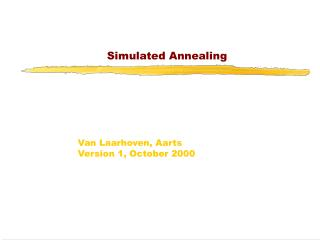DownloadDownload PresentationSimulated Annealing

# Simulated Annealing

Télécharger la présentation## Simulated Annealing

- - - - - - - - - - - - - - - - - - - - - - - - - - - E N D - - - - - - - - - - - - - - - - - - - - - - - - - - -
##### Presentation Transcript

1. Simulated Annealing Van Laarhoven, AartsVersion 1, October 2000

2. Iterative Improvement 1 • General method to solve combinatorial optimization problems Principle: • Start with initial configuration • Repeatedly search neighborhood and select a neighbor as candidate • Evaluate some cost function (or fitness function) and accept candidate if "better"; if not, select another neighbor • Stop if quality is sufficiently high, if no improvement can be found or after some fixed time

3. Iterative Improvement 2 Needed are: • A method to generate initial configuration • A transition or generation function to find a neighbor as next candidate • A cost function • An Evaluation Criterion • A Stop Criterion

4. Iterative Improvement 3 Simple Iterative Improvement or Hill Climbing: • Candidate is always and only accepted if cost is lower (or fitness is higher) than current configuration • Stop when no neighbor with lower cost (higher fitness) can be found Disadvantages: • Local optimum as best result • Local optimum depends on initial configuration • Generally no upper bound on iteration length

5. Hill climbing

6. How to cope with disadvantages • Repeat algorithm many times with different initial configurations • Use information gathered in previous runs • Use a more complex Generation Function to jump out of local optimum • Use a more complex Evaluation Criterion that accepts sometimes (randomly) also solutions away from the (local) optimum

7. Simulated Annealing Use a more complex Evaluation Function: • Do sometimes accept candidates with higher cost to escape from local optimum • Adapt the parameters of this Evaluation Function during execution • Based upon the analogy with the simulation of the annealing of solids

8. Other Names • Monte Carlo Annealing • Statistical Cooling • Probabilistic Hill Climbing • Stochastic Relaxation • Probabilistic Exchange Algorithm

9. Analogy • Slowly cool down a heated solid, so that all particles arrange in the ground energy state • At each temperature wait until the solid reaches its thermal equilibrium • Probability of being in a state with energy E : Pr { E = E } = 1/Z(T) . exp (-E / kB.T) E Energy T Temperature kB Boltzmann constant Z(T) Normalization factor (temperature dependant)

10. Simulation of cooling (Metropolis 1953) • At a fixed temperature T : • Perturb (randomly) the current state to a new state • E is the difference in energy between current and new state • If E < 0(new state is lower), accept new state as current state • If E  0, accept new state with probability Pr (accepted) = exp (- E / kB.T) • Eventually the systems evolves into thermal equilibrium at temperature T ; then the formula mentioned before holds • When equilibrium is reached, temperature T can be lowered and the process can be repeated

11. Simulated Annealing • Same algorithm can be used for combinatorial optimization problems: • Energy E corresponds to the Cost function C • Temperature T corresponds to control parameter c Pr { configuration = i } = 1/Q(c) . exp (-C(i) / c) C Cost c Control parameter Q(c) Normalization factor (not important)

12. Homogeneous Algorithm initialize; REPEAT REPEAT perturb ( config.i  config.j, Cij); IF Cij < 0 THEN accept ELSE IF exp(-Cij/c) > random[0,1) THEN accept; IF accept THEN update(config.j); UNTIL equilibrium is approached sufficient closely; c := next_lower(c); UNTIL system is frozen or stop criterion is reached

13. Inhomogeneous Algorithm • Previous algorithm is the homogeneous variant: c is kept constant in the inner loop and is only decreased in the outer loop • Alternative is the inhomogeneous variant: There is only one loop; c is decreased each time in the loop, but only very slightly

14. Parameters • Choose the start value of c so that in the beginning nearly all perturbations are accepted (exploration), but not too big to avoid long run times • The function next_lower in the homogeneous variant is generally a simple function to decrease c, e.g. a fixed part (80%) of current c • At the end c is so small that only a very small number of the perturbations is accepted (exploitation) • If possible, always try to remember explicitly the best solution found so far; the algorithm itself can leave its best solution and not find it again

15. Markov Chains 1 Markov Chain: Sequence of trials where the outcome of each trial depends only on the outcome of the previous one • Markov Chain is a set of conditional probabilities: Pij (k-1,k) Probability that the outcome of the k-th trial is j, when trial k-1 is i • Markov Chain is homogeneous when the probabilities do not depend on k

16. Markov Chains 2 • When c is kept constant (homogeneous variant), the probabilities do not depend on k and for each c there is one homogeneous Markov Chain • When c is not constant (inhomogeneous variant), the probabilities do depend on k and there is one inhomogeneous Markov Chain

17. Performance • SA is a general solution method that is easilyapplicable to a large number of problems • "Tuning" of the parameters (initial c, decrement of c, stop criterion) is relatively easy • Generally the quality of the results of SA is good, although it can take a lot of time • Results are generally notreproducible: another run can give a different result • SA can leave an optimal solution and not find it again(so try to remember the bestsolutionfoundsofar) • Proven to find the optimum under certain conditions; one of these conditions is that you must runforever